>  Test: BITSAT Mock Test- 1

# Test: BITSAT Mock Test- 1

Test Description

## 150 Questions MCQ Test BITSAT Mock Tests Series & Past Year Papers | Test: BITSAT Mock Test- 1

 1 Crore+ students have signed up on EduRev. Have you?
Test: BITSAT Mock Test- 1 - Question 1

### An electric motor operates at 20 r.p.s. What will be the approximate power delivered by the motor, if it supplies a torque of 75 N-m?

Test: BITSAT Mock Test- 1 - Question 2

### The main source of solar energy is

Test: BITSAT Mock Test- 1 - Question 3

### Three resistors are connected to form the sides of a triangle ABC, the resistance of the sides AB, BC and CA are 40 ohms, 60 ohms and 100 ohms respectively. The effective resistance between the points A and B in ohms will be

Detailed Solution for Test: BITSAT Mock Test- 1 - Question 3 Here resistor of side BC & AC are in series so
equ resis.= 100+60
= 160Ω
now this resis.is in parallel with resistance of AB
so1/rₑ= (1/40)+(1/160)
equ.resistance = 32
Test: BITSAT Mock Test- 1 - Question 4

A piece of Aluminium and Germanium each are cooled from T1 k to T2 k. The resistance of

Test: BITSAT Mock Test- 1 - Question 5

The ionisation potential of H-atom is 13.6V. When it is excited from ground state by monochromatic radiations of 970.6 Å, the number of emission lines will be (according to Bohr's theory)

Test: BITSAT Mock Test- 1 - Question 6

Which of the following statements is correct

Detailed Solution for Test: BITSAT Mock Test- 1 - Question 6
i) Photocell depends upon the amount of light falls on it, not on the frequency.

ii) The photocurrent is proportional to the intensity of incident light, not on the applied voltage.

iii) Photoelectric current increases with an increase in intensity.

iv) Stopping potential increases with increase in Kinetic energy which depends upon the frequency, not on the intensity.

So, the correct answer is (c).
Test: BITSAT Mock Test- 1 - Question 7

The number of turs of primary and secondary coils of a transformer are 5 and 10 respectively and mutual inductance of the transformer is 25 H. Now, number of turns in primary and secondary are made 10 and 5 respectuvely. Mutual inductance of transformer will be

Test: BITSAT Mock Test- 1 - Question 8

Threshold wavelength for photoelectric emission from a metal surface is 5200 Å. Photoelectrons will be emitted when this surface is illuminated with monochromatic radiation from

Detailed Solution for Test: BITSAT Mock Test- 1 - Question 8
Ans : (B)
For photoelectric emission to take place the wavelength of incident
light must be less than
threshold value which is 5200 A∘
wavelength of IR light = 7800 A∘
wavelength of UV light = 4000 A∘
Test: BITSAT Mock Test- 1 - Question 9

The capacitor of a parallel plate capacitor with no dielectric substance and a separation of 0.4 cm is 2 μF. The separation is reduced to half and it is filled with a substance of dielectric value 2.8. The new capacity of the capacitor is

Detailed Solution for Test: BITSAT Mock Test- 1 - Question 9

We know that capacitance, c = Kε0A / d
Thus we get when K = 1 and d = 0.4 we get c = 2F
Thus let the value of c when K = 2.8 and d = 0.2 be z
Thus we get z/2 = (1 / 0.4) / (2.8 / 0.2)
Thus we get z = 2 x 5.6 F
= 11.2 F

Test: BITSAT Mock Test- 1 - Question 10

Two concentric uniformly charged spheres of radius 5cm and 10cm are arranged as shown in the figure. Potential difference between the spheres is: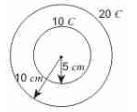Test: BITSAT Mock Test- 1 - Question 11

A block of mass 10 kg is placed on an inclined plane. When the angle of inclination is 30 o, the block just begins to side down the plane. The force of static friction is

Detailed Solution for Test: BITSAT Mock Test- 1 - Question 11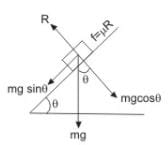Force of static friction f = μR
or f = mg sin θ, on point of sliding down of body.
∴ f = 10 x g x sin 30o
= 5(g) N = 5 kg wt.

Test: BITSAT Mock Test- 1 - Question 12

The angular velocity of rotation of star at which the matter strat to escape from its equator will be

Test: BITSAT Mock Test- 1 - Question 13

A perfect black body emits radiation at temperature 300 K. If it radiates 16 times this amount, then its temperature will be

Test: BITSAT Mock Test- 1 - Question 14

One litre of Helium gas at a pressure 76 cm of Hg and temperture 27o is heated till its pressure and volume are doubled. The final temperature attained by the gas is

Detailed Solution for Test: BITSAT Mock Test- 1 - Question 14
P1V1/T1=4P1V1/T2
T1=300K
THEN,
1/300=4/T2
T2=1200K
= 927degreeC
Test: BITSAT Mock Test- 1 - Question 15

If current in a moving coil galvanometer is increased by 1%, then percentage increase in its deflection will be

Test: BITSAT Mock Test- 1 - Question 16

A bar magnet is held perpendicular to a uniform magnetic field. If the couple acting on the magnet is to be halved by rotating it, then the angle by which it is to be rotated is

Test: BITSAT Mock Test- 1 - Question 17

What should be the minimum average velocity of water in a small tube of radius 5 mm, so that the flow is just turbulent? (Given: Viscosity of water=0.01 poise and density of water = 1000 kg-m⁻3)

Test: BITSAT Mock Test- 1 - Question 18

The neck and bottom of a bottle are 3 cm and 15 cm in radius respectively. If the cork is pressed with a force 12 N in the neck of the bottle, then force exerted on the bottom of the bottle is

Test: BITSAT Mock Test- 1 - Question 19

Two similar wires under the same load yield elongations of 0.1 mm and 0.05 mm respectively. If the area of cross-section of the first wire is 4 mm2, then the area of cross-section of the second wire is

Test: BITSAT Mock Test- 1 - Question 20

A stone is released from the top of a tower. If its velocity at half of the height is 10 m−s−1, then height of the tower is (Take g = 10 m−s−2)

Detailed Solution for Test: BITSAT Mock Test- 1 - Question 20 V² = 2as, = (10)² = 2*10*h/2 , ,,,,, and solve it, then, h = 10 m. Ans.
Test: BITSAT Mock Test- 1 - Question 21

For a particle in a uniformly accelerated circular motion

Test: BITSAT Mock Test- 1 - Question 22

The resultant of two forces acting at an angle of 150o is 10 N and is perpendicular to one of the forces. The other force is

Detailed Solution for Test: BITSAT Mock Test- 1 - Question 22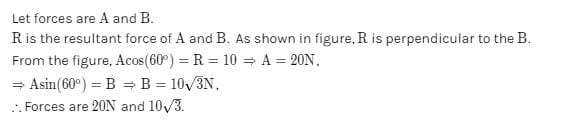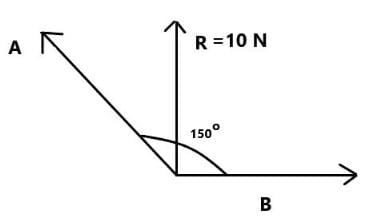Test: BITSAT Mock Test- 1 - Question 23

A body weighs 8 gm when placed in one pan and 18 gm when placed on the other pan of a false balance. If the beam is horizontal when both the pans are empty, the true weight of the body is

Detailed Solution for Test: BITSAT Mock Test- 1 - Question 23

If the beam is horizontal, the apparent weight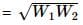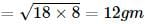Test: BITSAT Mock Test- 1 - Question 24

The length of a telescope is 36 cm. The focal lengths of its lenses can be

Detailed Solution for Test: BITSAT Mock Test- 1 - Question 24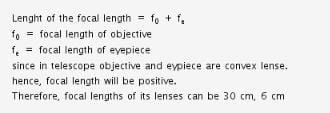Test: BITSAT Mock Test- 1 - Question 25

A mass M is suspended from the spring of negligible mass. The spring is pulled a little and then released, so that the mass executes simple harmonic motion of time period T. If the mass is increased by m, the time period becomes 5T/3. The ratio m/M is

Detailed Solution for Test: BITSAT Mock Test- 1 - Question 25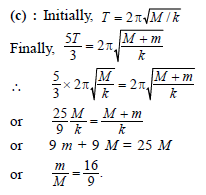Test: BITSAT Mock Test- 1 - Question 26

A body esecutes S.H.M. with amplitude A₀. At what displacement from the mean position, potential energy is one-fourth of its total energy?

Test: BITSAT Mock Test- 1 - Question 27

A compound microscope has two lenses. The magnifying power of one is 5 and the combined magnifying power is 100. The magnifying power of the other lens is

Test: BITSAT Mock Test- 1 - Question 28

A cylindrical solid of mass M has radius R and length L. Its moment of inertia about a generator is

Detailed Solution for Test: BITSAT Mock Test- 1 - Question 28

Explanation :  Generator of axis of a cylinder is a line lying on its surface and parallel to axis of cylinder

By parallel axis theorem

I = (MR2 ) /2 + MR2

= 3/2MR2

Test: BITSAT Mock Test- 1 - Question 29

Which of the following statements are correct when a gymnast sitting on a rotating stool with his arms outstretched, suddenly lowers his hands?

1. The angular velocity decreases.
2. The angular velocity increases.
3. The moment of inertia decreases.
4. The moment of inertia increases.
Select the answers using the codes given below.

Test: BITSAT Mock Test- 1 - Question 30

Two identical particles move towards eachother with velocity 2ν and ν respectively. The velocity of centre of mass is

Detailed Solution for Test: BITSAT Mock Test- 1 - Question 30 M(2v)-m(v)/m+m = v/2
Test: BITSAT Mock Test- 1 - Question 31

(1) The product of a volt and a coulomb is a joule.
(2) The product of a volt and an ampere is a joule/second
(3) The product of volt and watt is horse power.
(4) Watt-hour can be measured in terms of electron volt.
State if

Test: BITSAT Mock Test- 1 - Question 32

Which of the following is not thermodynamical function

Detailed Solution for Test: BITSAT Mock Test- 1 - Question 32 Work done by a system (W) = PdV. Therefore it is proportional to pressure and change in volume and does not depend upon the temperature. Therefore work done is not a thermodynamical function.
Test: BITSAT Mock Test- 1 - Question 33

Consider the following statements
Assertion (A) : In adiabatic compression, the internal energy and temperature of the system get decreased
Reason (R) : The adiabatic compression is a slow process
Of these statements

Test: BITSAT Mock Test- 1 - Question 34

In a circuit, the resistance is 10.845Ω and current is 3.23 A. Its potential difference in four significant figures after rounding off, will be

Test: BITSAT Mock Test- 1 - Question 35

If the ratio of intensities of two waves causing interference be 9:4, then the ratio of maximum and minimum intensities will be

Test: BITSAT Mock Test- 1 - Question 36

Doppler's effects in sound and light are

Detailed Solution for Test: BITSAT Mock Test- 1 - Question 36 Because sound waves are different than light waves.For sound waves, Doppler effect is dependent on both the speed of transmitter (something that is creating sound waves) and observer (something that is listening to that sound) but for light waves that is not the case since there is no medium needed.
Test: BITSAT Mock Test- 1 - Question 37

The first overtone in a closed pipe has a frequency

Test: BITSAT Mock Test- 1 - Question 38

If an open pipe of length l vibrates in fundamental mode, then pressure variation is maximum at

Detailed Solution for Test: BITSAT Mock Test- 1 - Question 38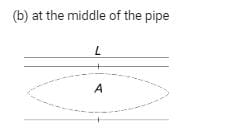For an open organ pipe in fundamental mode, an anti-node is formed at the middle, where the amplitude of the wave is maximum. Hence, the pressure variation is also maximum at the middle.
Test: BITSAT Mock Test- 1 - Question 39

The potential energy of a weight less spring compressed by a distance a is propotional to

Test: BITSAT Mock Test- 1 - Question 40

A ball moving with speed ν hits another identical ball at rest, The two balls stick together after collision. If specific heat of the material of the balls is S, the temperature rise resulting from the collision is

Detailed Solution for Test: BITSAT Mock Test- 1 - Question 40

By conservation of momentum, final velocity=v/2

And by energy conservation,

Initial K.E=Final K.E+heat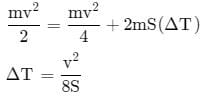Test: BITSAT Mock Test- 1 - Question 41

A compound is soluble in conc. H₂SO₄, it does not decolourise bromine in carbon tetrachloride but is oxidised by chromic anhydride in aqueous sulphuric acid within two seconds, turning orange solution to blue, green and then opaque. The original compound is

Test: BITSAT Mock Test- 1 - Question 42

Which of the following compounds does not react with NaHSO₃?

Detailed Solution for Test: BITSAT Mock Test- 1 - Question 42 All the aldehydes can smoothly react with sodiumbisuphite ,thus A and D are not the options now among ketones less sterically hindered ones can react with the sodiumbisuphite and thus C can react whereas B can't .Hopes it's clear .
Test: BITSAT Mock Test- 1 - Question 43

A 4f orbital has

Detailed Solution for Test: BITSAT Mock Test- 1 - Question 43

For 4f orbital n = 4, l = 4 – 1 = 3

Now, angular nodes = l = 3 and radial nodes = n – l – 1 = 0

Hence total nodes = 3

Test: BITSAT Mock Test- 1 - Question 44

The uncertainty principle and the concept of wave nature of matter was proposed by........and........respectively

Test: BITSAT Mock Test- 1 - Question 45

Molarity of liquid HCl with density equal to 1.17 g/cc is

Detailed Solution for Test: BITSAT Mock Test- 1 - Question 45
Given:
Density of HCl=1.17g/cm^3
Molarity=(density/molar mass)x1000 moles per liter
molar mass of HCl=[1+35.5]=36.5g/mol
Molarity=(1.17/36.5)x1000 =32.05M
∴Molarity of liquid HCl with density equal to 1.17 g/cc is 32.05

Test: BITSAT Mock Test- 1 - Question 46

Which of the following has the smallest number of molecules?

Detailed Solution for Test: BITSAT Mock Test- 1 - Question 46
0.1 mol CO2 = 0.1 Na molecules
11.2 litres CO2 = 0.5 Na molecules
22 gm CO2 = 0.5 Na molecules
22.4 * 10^3 ml CO2 = 1 Na molecules
Hence
Option A is correct
Test: BITSAT Mock Test- 1 - Question 47

Which of the following is an electrophile?

Test: BITSAT Mock Test- 1 - Question 48

Electrophiles are

Detailed Solution for Test: BITSAT Mock Test- 1 - Question 48 Electrophiles are electron loving chemical species. Their attraction for electrons is due to the presence of an electron deficient atom in them. Electrophiles may be either positively charged or electrically neutral chemical species i.e.
Test: BITSAT Mock Test- 1 - Question 49

An example of water soluble vitamin is

Detailed Solution for Test: BITSAT Mock Test- 1 - Question 49 In contrast, water-soluble vitamins dissolve in water and are not stored by the body. Since they are eliminated in urine, we require a continuous daily supply in our diet. The water-soluble vitamins include the vitamin B-complex group and vitamin C.Water-soluble vitamins are easily destroyed or washed out during food storage or preparation. Proper storage and preparation of food can minimize vitamin loss. To reduce vitamin loss, always refrigerate fresh produce, keep milk and grains away from strong light, and use cooking water from vegetables to prepare soups.
Test: BITSAT Mock Test- 1 - Question 50

Phospholipids are esters of glycerol with

Test: BITSAT Mock Test- 1 - Question 51

Electrolysis of aqueous solution of CH₃COOK gives

Test: BITSAT Mock Test- 1 - Question 52

Which pair of species given below have identical bond order?

Test: BITSAT Mock Test- 1 - Question 53

For a reaction to occur spontaneously,

Detailed Solution for Test: BITSAT Mock Test- 1 - Question 53 ∆G=∆H-T∆S if ∆G is negative then the rxn is spontaneous.
Test: BITSAT Mock Test- 1 - Question 54

A and B are gaseous substances which react reversibly to give two gaseous substances C and D,accompanied by the liberation of heat.When the reaction reaches equilibrium,it is observed that Kp = Kc.The equilibrium cannot be disturbed by

Detailed Solution for Test: BITSAT Mock Test- 1 - Question 54

Explanation : A+B---> C+D

moles of reactant = 1+1 = 2

moles of product = 1+1 = 2

Suppose pressure is increased double, then volume will decrease to half

Let initial volume as V

final volume as V/2

k[initial] = [C][D]/[A][B]

= (nC/V*nD/V)/(nA/V*nB/V)

= (nCnD)/(nAnB)

k[final] = [C][D]/[A][B]

= (nc/(V/2)*nD/(V/2))/(nA/(V/2)*nB/(V*2))

= (ncnD)/(nAnB)

Test: BITSAT Mock Test- 1 - Question 55

The rate of chemical reaction is doubled for every 10oC rise in temperature because of

Test: BITSAT Mock Test- 1 - Question 56

Which of the following has the highest electronegativity?

Detailed Solution for Test: BITSAT Mock Test- 1 - Question 56

Electronegativity of an element is the tendency of its atom to attract the shared pair of electrons towards itself in a covalent bond. The elements of highest electronegativity in the periodic table are:
F (4.0) > O (3.5) > Cl (3.0) = N (3.0) .

Test: BITSAT Mock Test- 1 - Question 57

Standard enthalpy and standard entropy changes for the oxidation of ammonia at 298 K are - 382.64 kJ mol-1 and - 145.6 JK-1 mol-1 respectively. The standard Gibbs energy change for the same reaction 298 K is

Detailed Solution for Test: BITSAT Mock Test- 1 - Question 57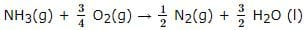T = 298 K
ΔH = - 382.64 kJ mol-1
ΔS = - 145.6 JK-1 mol-1
= - 0.1456 kJ K-1 mol-1
ΔG = ΔH - TΔS
= -382.64 kJ mol-1 - (298 K) x (0.1456 k JK-1 mol-1)
= -382.64 kJ mol-1 + 43.3888 kJ mol-1
= -339.25 kJ mol-1 ≌ 339.3 kJrnol-1

Test: BITSAT Mock Test- 1 - Question 58

Aspirin is an acetylation product of

Detailed Solution for Test: BITSAT Mock Test- 1 - Question 58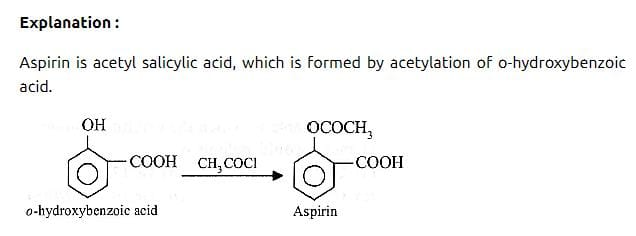Test: BITSAT Mock Test- 1 - Question 59

Which of the following complexes will have four isomers?

Detailed Solution for Test: BITSAT Mock Test- 1 - Question 59

(a) will have four different types of isomers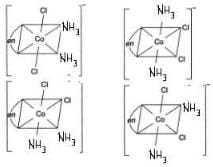Test: BITSAT Mock Test- 1 - Question 60

The lanthanide contraction is responsible for the fact that

Test: BITSAT Mock Test- 1 - Question 61

Manganese belongs to

Test: BITSAT Mock Test- 1 - Question 62

When EoAg⁺/Ag = 0.80 V and EZn2⁺/Zn = - 0.76 V which of the following is correct ?

Test: BITSAT Mock Test- 1 - Question 63

Octane number is zero for

Detailed Solution for Test: BITSAT Mock Test- 1 - Question 63 The octane number is based on an arbitrary scale with zero assigned to n-heptane and 100 to 2,2,4-trimethylpentane (isooctane). Mixtures of both alkanes exhibit octane numbers between 0 and 100 depending on the ratio of the mixture.
Test: BITSAT Mock Test- 1 - Question 64

2-Methylbutane on reacting with bromine in the presence of sunlight gives mainly

Test: BITSAT Mock Test- 1 - Question 65

Which of the following on oxidation gives H₂O₂?

Test: BITSAT Mock Test- 1 - Question 66

When of the following statements is correct ?

Test: BITSAT Mock Test- 1 - Question 67

Which of the following has chiral carbon atom?

Test: BITSAT Mock Test- 1 - Question 68

Among the following compounds the one with the highest percentage of chlorine is

Detailed Solution for Test: BITSAT Mock Test- 1 - Question 68 Chemical formula of pyrene is CCl4
in this compound according to mass 97.5 percent chlorine is found.
Test: BITSAT Mock Test- 1 - Question 69

Oxalic acid reacts with concentrated H₂SO₄ to give a mixture of two gases. When this mixture is passed through caustic potash, one of the gases is absorbed. What is the product formed by the absorbed gas with caustic potash?

Test: BITSAT Mock Test- 1 - Question 70

On heating a solution of NH₄Cl and KNO2, we get

Detailed Solution for Test: BITSAT Mock Test- 1 - Question 70

On heating mixture of NH4​Cl and KNO2​, N2​ is obtained with potassium chloride and water.

NH4​Cl+KNO2​⟶N2​+KCl+2H2​O

Test: BITSAT Mock Test- 1 - Question 71

Which one of the following properties is not correct for ozone?

Test: BITSAT Mock Test- 1 - Question 72

BF₃ is used as a catalyst in several industrial processes due to its

Detailed Solution for Test: BITSAT Mock Test- 1 - Question 72

The boron trihalides are covalent in nature and exist as monomeric molecules having planar triangular geometry. In these halides, boron assumes sp2 hybrid state and the three B−X bonds are formed by the axial overlap of sp2 hybrid orbital of boron and p-orbital of halogen as shown in Fig.  Since there are only six electrons in the valence shell of a boron atom in boron trihalides, therefore, they have a great tendency to accept two more electrons in order to acquire stable octet.

Hence, boron trihalides act as Lewis acids. They rapidly combine with electron donors such as NH3​, F−ion, amines, ether, sulphides or phosphorus.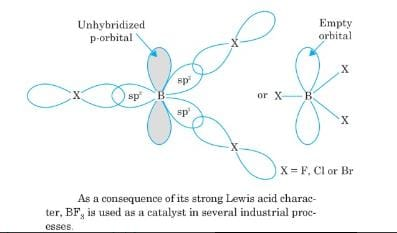Test: BITSAT Mock Test- 1 - Question 73

Nitrous oxide is

Detailed Solution for Test: BITSAT Mock Test- 1 - Question 73 At room temperature, it is a colourless non-flammable gas, with a slight metallic scent and taste. At elevated temperatures, nitrous oxide is a powerful oxidizer similar to molecular oxygen. It is soluble in water.
Test: BITSAT Mock Test- 1 - Question 74

Which of the following polymer is prepared from single monomer?

Detailed Solution for Test: BITSAT Mock Test- 1 - Question 74 Nylon-6 is made from a single monomer called Caprolactam.
Test: BITSAT Mock Test- 1 - Question 75

Which one of the following compounds liberates CO2 from aqueous NaHCO3

Test: BITSAT Mock Test- 1 - Question 76

In Solvay process of manufacture of Na₂CO₃, the by products are

Test: BITSAT Mock Test- 1 - Question 77

The molarity of a solution of Na₂CO₃ having 10.6 g/500 ml of solution is

Detailed Solution for Test: BITSAT Mock Test- 1 - Question 77
Molarity can be found with this formula:

molarity=number of moles/volume (L)

We know the volume, which is

500mL = 0.5 L.

We still need to know the number of moles, though, but we can find that from its mass.

10.6 g of Na2CO3 is:

10.6g molar mass=10.6g/106 g/mol=0.1 mol

Now, we can just plug those values in to solve for molarity:

molarity=0.1 mol/0.5 L=0.2 M
Test: BITSAT Mock Test- 1 - Question 78

In crystal structure of sodium chloride, the arrangement of Cl⁻ ion is

Test: BITSAT Mock Test- 1 - Question 79

12 litre of hydrogen and 11.2 litre of chlorine are mixed and exploded. The composition in volume of the mixture will be

Test: BITSAT Mock Test- 1 - Question 80

Alum helps in purifying water by

Test: BITSAT Mock Test- 1 - Question 81

The area bounded by the parabola x = 4 - y2 and the y-axis in square units, is

Test: BITSAT Mock Test- 1 - Question 82

In the expansion of (1+x+x2+x3)5 the sum of coefficients of even powers of x is :

Test: BITSAT Mock Test- 1 - Question 83

The equation of the circle when touches x-axis at (3,0) and passing through (1,4) is

Detailed Solution for Test: BITSAT Mock Test- 1 - Question 83 Since the circle touches the x axis the y coordinate of center is equal to the radius

Let the equation of the circle be x² + y² -2hx -2ky+ c = 0

r² = h² + k² - c

But r = k

h² = c

Eq of circle
x² + y² -2hx-2ky + h² = 0

Circle passes through (3,0)

9 + 0 -6h + 0 +h²= 0

(h - 3)² = 0

h = 3

c = 9

Eq of circle
x² + y² -6x - 2ky + 9 = 0

Circle passes through (1,4)
1 + 16 - 6 - 8k + 9 = 0

8k = 20
k = 5/2

Therefore eq of circle

x² + y² -6x -5y + 9 = 0
Test: BITSAT Mock Test- 1 - Question 84

If r = 2a cosθ represents a circle, then its centre is

Test: BITSAT Mock Test- 1 - Question 85

If z = [(1-i√3)/(1+i√3)], then arg (z) =

Detailed Solution for Test: BITSAT Mock Test- 1 - Question 85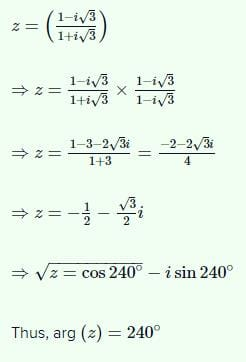Test: BITSAT Mock Test- 1 - Question 86

The solution of the equation y - x(dy/dx) = a[(y2)+(dy/dx)] is

Test: BITSAT Mock Test- 1 - Question 87

The differential equation for the family of curves x2+ y2 - 2ay = 0, where a is an arbitrary constant is

Test: BITSAT Mock Test- 1 - Question 88

The differential equation of family of curves y = a cos(x+b) is

Test: BITSAT Mock Test- 1 - Question 89

In which interval function f(x)=2x3-15x2+36x+1 is decreasing

Test: BITSAT Mock Test- 1 - Question 90

If y=log logx, ey(dy/dx)=

Test: BITSAT Mock Test- 1 - Question 91

Find the sum of the series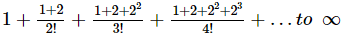Detailed Solution for Test: BITSAT Mock Test- 1 - Question 91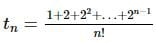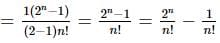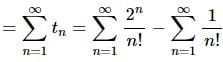Sum of the given series
= (e2 - 1) - (e - 1) = e2 - e = e (e - 1)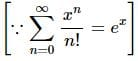Test: BITSAT Mock Test- 1 - Question 92

The tangents to the hyperbola x2 - y2 = 3 are parallel to the st. line 2x + y + 8 = 0 at the following points

Test: BITSAT Mock Test- 1 - Question 93

A flag staff 5m high is placed on a building 25m high. If flag and building both subtend equal angles on the observer at a height 30m, the distance between the observer and the top of the flag is

Detailed Solution for Test: BITSAT Mock Test- 1 - Question 93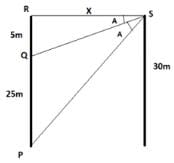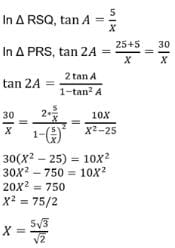Test: BITSAT Mock Test- 1 - Question 94

The area between (x2/a2) + (y2/b2) = 1 and the straight line (x/a) + (y/b) = 1 is

Detailed Solution for Test: BITSAT Mock Test- 1 - Question 94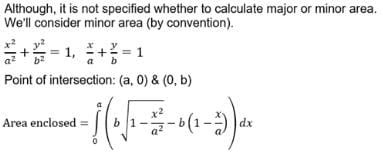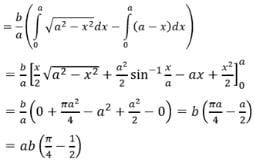Test: BITSAT Mock Test- 1 - Question 95

Solution of the equation (x + 2y3) (dy/dx) - y = 0 is (y > 0)

Test: BITSAT Mock Test- 1 - Question 96

The sum of first 50 terms of the series cot⁻1 3 + cot⁻1 7 + cot⁻1 13 + cot⁻1 21 + ... is

Test: BITSAT Mock Test- 1 - Question 97

The period of the function f(x) = sin(πx/2)+2 cos(πx/3)-tan(πx/4) is

Test: BITSAT Mock Test- 1 - Question 98

If Sn = 13 + 23 + … + n3 and Tn = 1 + 2 + … + n then

Detailed Solution for Test: BITSAT Mock Test- 1 - Question 98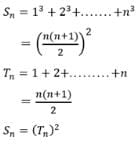Test: BITSAT Mock Test- 1 - Question 99

If A and B are squares matrices of the same type then

Test: BITSAT Mock Test- 1 - Question 100

If in a square matrix A=[aij], we find that
aij = aji ∀ i,j, then A is a

Test: BITSAT Mock Test- 1 - Question 101

The point on the hyperbola (x2/24)-(y2/18)=1 which is nearest to the line 3x+2y+1=0 is

Test: BITSAT Mock Test- 1 - Question 102

The number log₂ 7 is

Detailed Solution for Test: BITSAT Mock Test- 1 - Question 102 Lets assume that √7 is rational number. ie √7=p/q. we divide by the common factor to get √7 = a/b were a and b are co-prime number. that is a and b have no common factor.
Test: BITSAT Mock Test- 1 - Question 103

If angle between the lines 2x2+5xy+3y2+6x+7y+4=0 is tan⁻1m, the value of m is

Detailed Solution for Test: BITSAT Mock Test- 1 - Question 103 The angle between the pair of straight lines is given by

Theta = tan¹|(2√h²-ab)/a + b| = tan¹m

2√h² - ab/a + b. = m

a = 2, b = 3, h= 5/2

2√25/4 - 6/ 5 = m

2(√1/4) /5 = m

m = 1/5
Test: BITSAT Mock Test- 1 - Question 104

The tangents at the points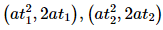on the parabola y2 = 4 a x are at right angles if

Test: BITSAT Mock Test- 1 - Question 105

The length of the normal chord to the parabola y2 = 4x which subtends a right angle at the vertex is

Test: BITSAT Mock Test- 1 - Question 106

How many even numbers can be formed by using all the digits 2, 3, 4, 5, 6?

Test: BITSAT Mock Test- 1 - Question 107

There are 16 points in a plane in which 6 are collinear. How many lines can be drawn by joining these points?

Test: BITSAT Mock Test- 1 - Question 108

A box contains 10 good articles and 6 with defects. One item is drawn at random, what is the probability that it is either good or has a defect, is :

Test: BITSAT Mock Test- 1 - Question 109

In eight throws of 1 die or 3 is considered a success. Then the standard deviation of the success is

Detailed Solution for Test: BITSAT Mock Test- 1 - Question 109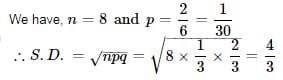Test: BITSAT Mock Test- 1 - Question 110

In a triangle the line joining circum-centre and in-centre is parallel to BC, then cosB+cosC=

Test: BITSAT Mock Test- 1 - Question 111

If R denotes circumradius then in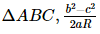is equal to

Detailed Solution for Test: BITSAT Mock Test- 1 - Question 111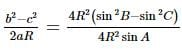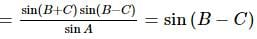Test: BITSAT Mock Test- 1 - Question 112

f(x) = 1 + 2sinx + 3cos2x, 0≤x≤(2π/3) is

Test: BITSAT Mock Test- 1 - Question 113

The sum of first n terms of the series (3/12) + (5/(12 + 22)) + (7/(12 + 22 + 32)) + ... is

Test: BITSAT Mock Test- 1 - Question 114

Every term of a G.P. is positive and also every term is the sum of two preceding terms. Then the common ratio of the G.P. is

Test: BITSAT Mock Test- 1 - Question 115

Let f (x) = (sin x)/(1 + √sinx). If D is the domain of f, then D contains

Test: BITSAT Mock Test- 1 - Question 116

If f (x) = (x)/(x - 1), then f (a)/f (a + 1) =

Detailed Solution for Test: BITSAT Mock Test- 1 - Question 116

f(x)=x/(x-1)
f(x+1)=(x+1)/x  (Put x = x+1)

Divide f(x) by f(x+1), we get => x^2 / (x^2 - 1)
Hence  f(a)/f(a+1)= a^2 / (a^2 - 1) = f(a^2)

Test: BITSAT Mock Test- 1 - Question 117

The equation of line passing through lines 5x-6y-1=0 and 3x+2y+5=0 and perpendicular to 3x-5y+11=0 is

Test: BITSAT Mock Test- 1 - Question 118

The slope of the tangent of the locus y = cos-1 (cos x) at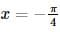is

Test: BITSAT Mock Test- 1 - Question 119

The direction cosines of a line equally inclined to the +ve directions of axes are

Test: BITSAT Mock Test- 1 - Question 120

The centre of the sphere which passes through' four points (0,0,0),(0,2,0),(1,0,0) and (0,0,4) is

Test: BITSAT Mock Test- 1 - Question 121

If tan(πcosθ)=cot(πsinθ), then cos[(θ)-(π/4)] =

Test: BITSAT Mock Test- 1 - Question 122

The general value of θ which satisfies the equations sinθ = -(1/2) and tanθ = (1/√3) is

Detailed Solution for Test: BITSAT Mock Test- 1 - Question 122 Hence in third quodrant sin-1/2 and tan1√3 are common so
angle (thitha)=210
in π term thitha=210×π/180=7π/6
thitha=2nπ+alpha
here alpha=7π/6
so thitha=2nπ+7π/6
Test: BITSAT Mock Test- 1 - Question 123

The value of cos 1ocos2ocos3o...cos179o is equal to

Test: BITSAT Mock Test- 1 - Question 124

If p=7i-2j+3k and q=3i+j+5k, then the modulus of p-2q is

Detailed Solution for Test: BITSAT Mock Test- 1 - Question 124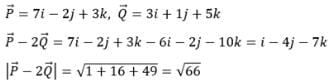Test: BITSAT Mock Test- 1 - Question 125

Find the antonym of NETTLE.

Test: BITSAT Mock Test- 1 - Question 126

If θ is the angle between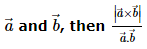equals

Detailed Solution for Test: BITSAT Mock Test- 1 - Question 126 |a × b| =| a|.|b| sin(theta)

a. b =| a||b|cos(theta)

|a × b| / a. b = |a||b|sin(theta) / |a||b|cos(theta)

= tan(theta)
Test: BITSAT Mock Test- 1 - Question 127

Find the Antonym of the word occurring in the sentence in capital letter as per the context.
For the first time I saw him speaking RUDELY to her

Detailed Solution for Test: BITSAT Mock Test- 1 - Question 127

'Rudely' means showing lack of respect.
So its antonym will be Politely

Test: BITSAT Mock Test- 1 - Question 128

Fill in the blank with appropriate word.
It is difficult to believe what he tells us because his account of any event is always full of......of all sorts.

Test: BITSAT Mock Test- 1 - Question 129

Fill in the blank with appropriate word.
Joseph introduced me .......... his mother as the best batsman.

Test: BITSAT Mock Test- 1 - Question 130

Choose the one which can be substituted for the given words/sentences
A style full of words.

Detailed Solution for Test: BITSAT Mock Test- 1 - Question 130
Pedantic means one who speak elaborately the small thing. That is he concern more for even small thing.
Verbose means one who speak huge vocabulary and unnecessary words to making stylish his speech.
Test: BITSAT Mock Test- 1 - Question 131

Choose the one which can be substituted for the given words/sentences
To take secretly in small quantities

Test: BITSAT Mock Test- 1 - Question 132

Spot the error.

Test: BITSAT Mock Test- 1 - Question 133

Spot the error.

Test: BITSAT Mock Test- 1 - Question 134

Spot the error.

Detailed Solution for Test: BITSAT Mock Test- 1 - Question 134

So that he might top the list

Test: BITSAT Mock Test- 1 - Question 135

Find the Synonym of the word occurring in the sentence in capital letter as per the context.
Very few people are IMPERVIOUS to criticism.

Detailed Solution for Test: BITSAT Mock Test- 1 - Question 135

'Impervious' means inaccessible.
In the current context, the question word means 'not disturbed by'
Hence the correct answer options is Unaffected

Test: BITSAT Mock Test- 1 - Question 136

Find the Synonym of the word occurring in the sentence in capital letter as per the context.
Her habits are INNOCUOUS.

Detailed Solution for Test: BITSAT Mock Test- 1 - Question 136

'Innocuous' means not harmful or dangerous

Test: BITSAT Mock Test- 1 - Question 137

Choose the pair in which the words are differently related .

Detailed Solution for Test: BITSAT Mock Test- 1 - Question 137

Except Water : Tap
In all other pairs, second requires the first to function

Test: BITSAT Mock Test- 1 - Question 138

Complete the analogous pair.
Gun : Bullet : : Chimney : ?

Detailed Solution for Test: BITSAT Mock Test- 1 - Question 138 Second comes out of the first.Correct option is D.
Test: BITSAT Mock Test- 1 - Question 139

Group the following figures into three classes on the basis of identical properties.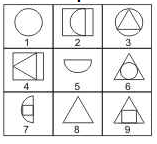Detailed Solution for Test: BITSAT Mock Test- 1 - Question 139

1,3,6 contain one complete circle each.
2,5,7 contain a semi-circle each.
4,8,9 contain a triangle each.

Test: BITSAT Mock Test- 1 - Question 140

Find the missing character.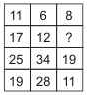Detailed Solution for Test: BITSAT Mock Test- 1 - Question 140

In the first column, 17-11=25-19=6.
In the second column, 12-6=34-28=6.
Let the missing number in the third column be x.
Then, x-8=19-11=8 or x=16.

Test: BITSAT Mock Test- 1 - Question 141

Find out the term in the number series which is wrong.
11, 2, 21, 3, 32, 4, 41, 5, 51, 6

Detailed Solution for Test: BITSAT Mock Test- 1 - Question 141

The given sequence is a combination of two series :
I. 11, 21, 32, 41, 51
II. 2, 3, 4, 5, 6.
The pattern in I is + 10.
So, 32 is wrong and should be replaced by (21 + 10) i.e. 31

Test: BITSAT Mock Test- 1 - Question 142

In this letter series, some of the letters are missing. Choose the correct letter given below -
b _ ac _ cc _ cb _ ab _ ac

Test: BITSAT Mock Test- 1 - Question 143

Jogging, or running at an easy pace, is really popular now. It's fun, free and a quick way to get fit. It's very good for suppleness or upper body strength.
There's some risk of overuse injury to feet, knees, ankles and hips. But if you don't overdo it at first, and run on soft surface like grass when you can, you shouldn't have serious problems. If you have arthritis in your legs, hips or back or if you are overweight, try cycling or swimming instead.
The only expense is a good pair of running shoes. Avoid busy roads and jogging at night if possible. Wear light-coloured clothes so as not to feel uneasy and a cyclist's reflective chest band.

Q. Jogging is a popular exercise because it

Test: BITSAT Mock Test- 1 - Question 144

Jogging, or running at an easy pace, is really popular now. It's fun, free and a quick way to get fit. It's very good for suppleness or upper body strength.
There's some risk of overuse injury to feet, knees, ankles and hips. But if you don't overdo it at first, and run on soft surface like grass when you can, you shouldn't have serious problems. If you have arthritis in your legs, hips or back or if you are overweight, try cycling or swimming instead.
The only expense is a good pair of running shoes. Avoid busy roads and jogging at night if possible. Wear light-coloured clothes so as not to feel uneasy and a cyclist's reflective chest band.

Q. Sometimes jogging can become risky when

Test: BITSAT Mock Test- 1 - Question 145

Jogging, or running at an easy pace, is really popular now. It's fun, free and a quick way to get fit. It's very good for suppleness or upper body strength.
There's some risk of overuse injury to feet, knees, ankles and hips. But if you don't overdo it at first, and run on soft surface like grass when you can, you shouldn't have serious problems. If you have arthritis in your legs, hips or back or if you are overweight, try cycling or swimming instead.
The only expense is a good pair of running shoes. Avoid busy roads and jogging at night if possible. Wear light-coloured clothes so as not to feel uneasy and a cyclist's reflective chest band.

Q. Jogging is not advised for people who

Test: BITSAT Mock Test- 1 - Question 146

Jogging, or running at an easy pace, is really popular now. It's fun, free and a quick way to get fit. It's very good for suppleness or upper body strength.
There's some risk of overuse injury to feet, knees, ankles and hips. But if you don't overdo it at first, and run on soft surface like grass when you can, you shouldn't have serious problems. If you have arthritis in your legs, hips or back or if you are overweight, try cycling or swimming instead.
The only expense is a good pair of running shoes. Avoid busy roads and jogging at night if possible. Wear light-coloured clothes so as not to feel uneasy and a cyclist's reflective chest band.

Q. Everybody can afford to jog as it

Test: BITSAT Mock Test- 1 - Question 147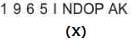Test: BITSAT Mock Test- 1 - Question 148

Select the correct mirror image of the figure (X) from amongst the given alternatives.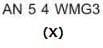Test: BITSAT Mock Test- 1 - Question 149

Each of the following questions consists of five Problem figures marked 1,2,3,4 and 5 followed by five Answer figures marked A,B,C,D and E . Select a figure from the Answer figures which will continue the same series as given in the Problem figures.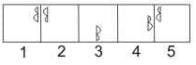Test: BITSAT Mock Test- 1 - Question 150

Each of the following questions consists of five Problem figures marked 1,2,3,4 and 5 followed by five Answer figures marked A,B,C,D and E . Select a figure from the Answer figures which will continue the same series as given in the Problem figures.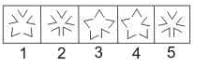## BITSAT Mock Tests Series & Past Year Papers

2 videos|15 docs|70 tests
 Use Code STAYHOME200 and get INR 200 additional OFF Use Coupon Code

## BITSAT Mock Tests Series & Past Year Papers

2 videos|15 docs|70 tests